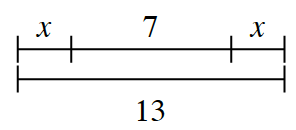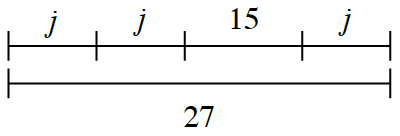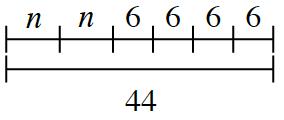### Home > CC2 > Chapter Ch6 > Lesson 6.2.6 > Problem6-127

6-127.

Each of the diagrams below represents a sequence for an acrobat on a tightrope. Each letter represents the unknown length of a trick. For each part below, write and solve an equation to figure out how far the acrobat travels during each trick (that is, the length represented by each letter). Show how you know your answer is correct.

1. Find $x$.If $x+7+x$ is the same as $13$, can you think of a way to find the value of $x$?

To find the value of both $x$'s combined, you can subtract $7$ from $13$.
$13-7=6$

Now you know that two $x$'s are equal to $6$. Can you find the value of one $x$?

1. Find $j$.Do you think you can use a similar strategy as in part (a)? Remember, try to find the value of just one $j$.

$27$ is the same as $j+j+15+j$. Can you find the value of $j$?

$j=4$. Remember to show how you know!

1. Find $n$.Remember that $6+6+6+6$ is the same as $6(4)$. This simplification should make finding $n$ easier.

If two $n$'s equal $20$, how much is one $n$?